# Socially Distant Polygons

Imagine a polygon, like the blue one below. Where should you stand in that polygon such you are as far as possible from your nearest vertex? This seems like a useful thing to be able to calculate in 2020 (hope this blog post ages well). Ostensibly this should be easy to compute too, but it’s not obviously that the red dot in the image below is in fact the furthest point from any of the polygon vertices.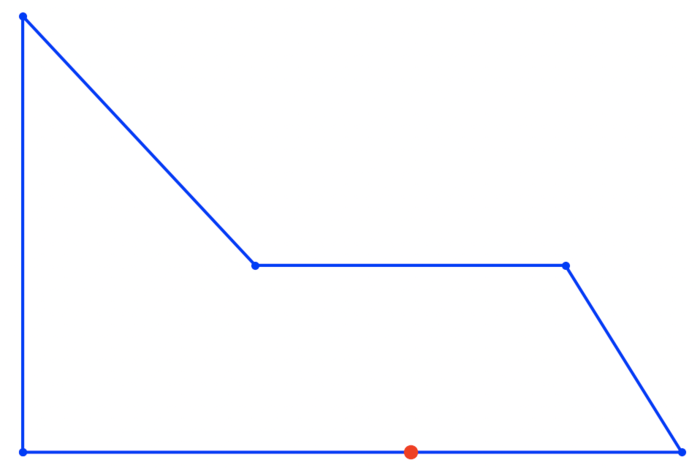A first guess might be to stand half the longest edge length away from one of the vertices. But unfortunately that doesn’t work. If you try a square you’ll see it’s best to stand in the centre which is slightly further away from each corner. This shows that we need to consider points on the interior of the polygon to make sure they are covered too. Stated another way we are trying to calculate the minimum radius circle `R`, such that the area of the union of circles positioned at `(xi, yi)` the vertices each having `R` have the same area as the polygon `P`. If the radius is too small the polygon won’t be covered and just as the area completely coverage the polygon. That very last point is the point we are searching for.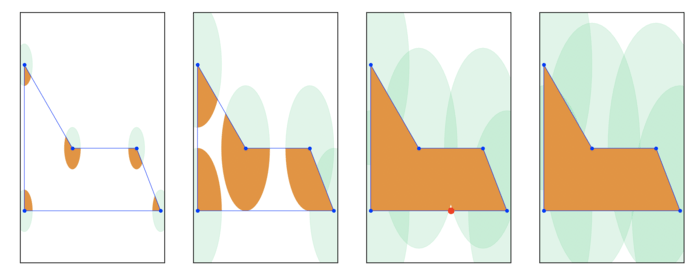If we write this area of the union or the circle as a function of `R` then `UnionArea(R)` will be monotonic, namely if `R1 < R2`, then `UnionArea(R1) <= UnionArea(R2)`. This nice yields a short solution, where we try increasing values of `R` until the `UnionArea(R) == Area(P)`. Keep increasing `R` until we’ve filled up the polygon with orange (union of circle) and as so as we have done so, stop.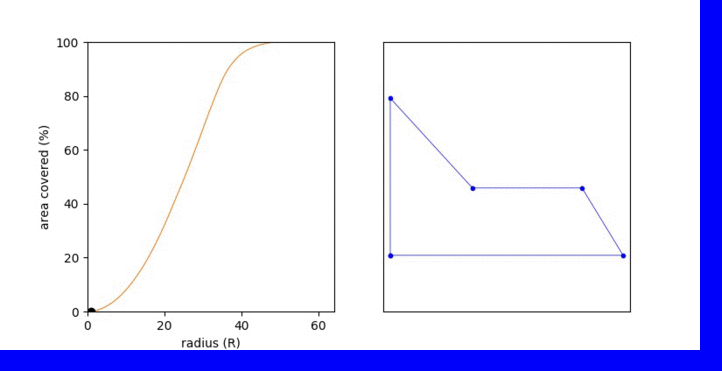Knowing the function is monotonic we can do a binary search over the range `R = {0 .. MAX}`, and we want the smallest value of `R` which covers 100% of the polygon.

This is easy enough to code but computing the union of thousands of circles gets very complicated and slows things down. Although it’s short to code using a computational geometry library, this solution quickly falls over for polygons like this horror: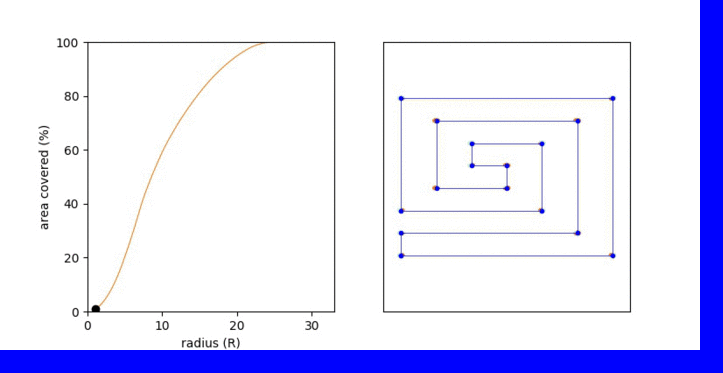Let’s look at this again. We are searching for the point inside the polygon that is further away from any vertex. If we can construct a region around each vertex where all points inside that region are nearest to that vertex then the furthest point is going to lie somewhere on the boundary of those regions.

## Voronoi Diagrams #

The problem of decomposing space into regions containing a set of points such that each point in a region is nearest to that point is called a Voronoi decomposition. Here’s what it looks like for a polygon’s vertices.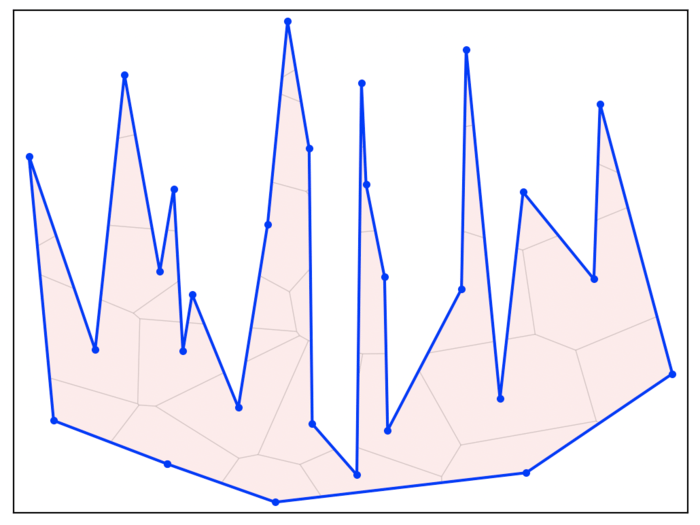So let’s try and compute the Voronoi diagram for a set of points. A naive approach is to iterate through each point, then for each other point we find the perpendicular bisector which delineates a half plane, collecting the union of these half planes gives us these regions around each point. In the following image region is being constructed around the red vertices. The green points are the midpoint of the red vertex with all the other vertices of the polygon. These lie on the perpendicular bisector that delineates the half-planes which are show in green. Any point that isn’t in one of these half-planes is nearer to the red vertex than any other vertex. This is the red region, in this case only shown inside the polygon.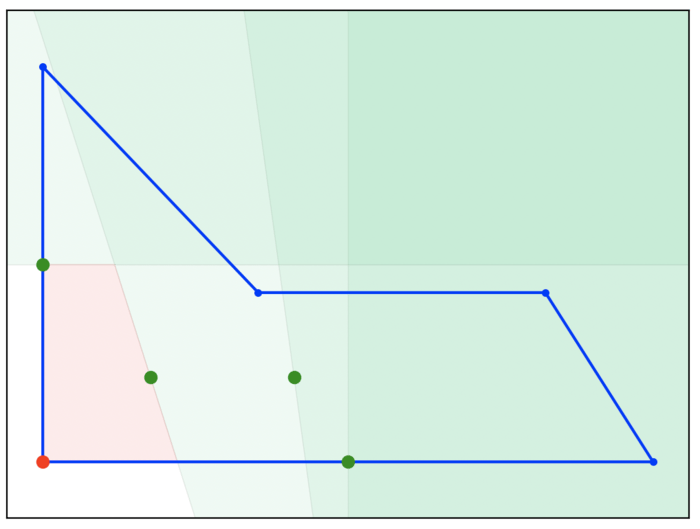Performing this operation for all vertices of the polygon will give the decomposition of space within the polygon which looks like this. Again any point inside a red region is nearer to the polygon vertex inside that same region than any other polygon vertex.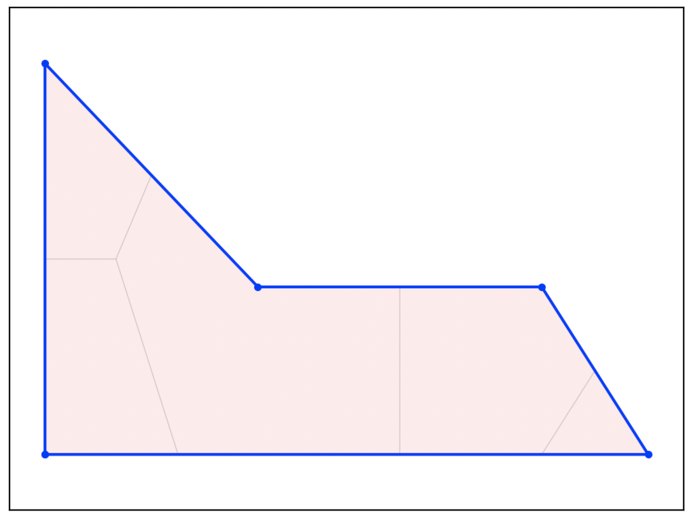This works well but again we are maintaining a growing set of half-planes, which while simpler than the circle still gets too complicated: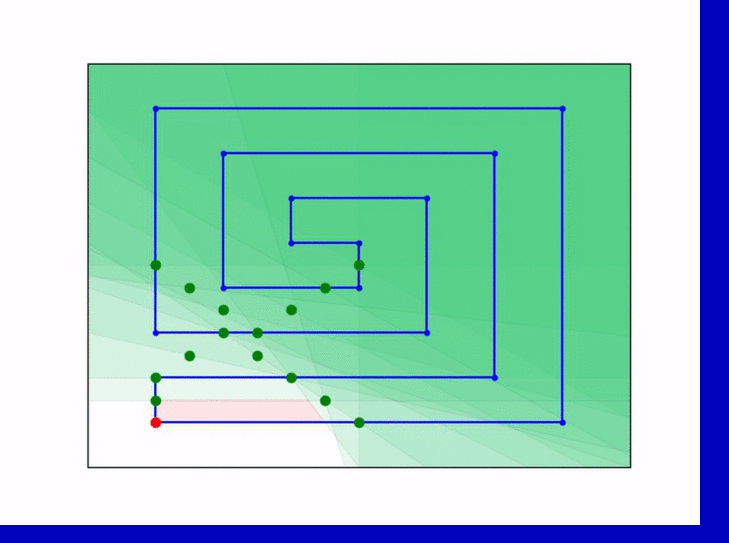Which yields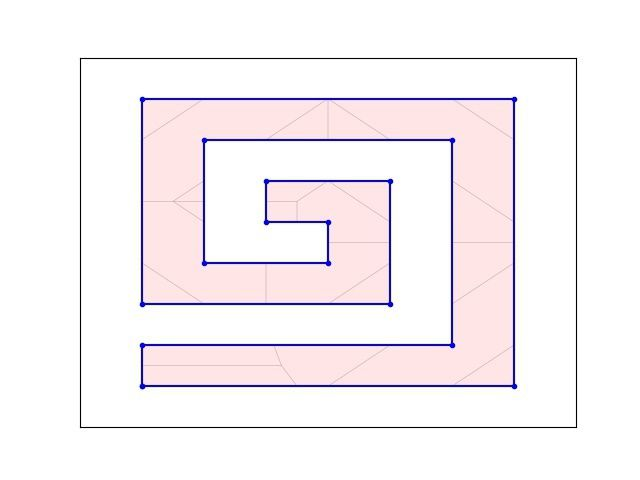Possibly the best way to compute the Voronoi diagram is to use Fortune’s algorithm. It is such an elegant algorithm to watch as it directly traces out the regions while sweeping from bottom to top. This is what I mean! Fortune’s algorithm works but sorting the vertices from bottom to top and processing them in order. While doing so it maintains a piece-wise parabola that looks like the beach which describes regions nearest to the points currently under consideration. The algorithm uses a balanced tree structure to maintain the beach line and a priority queue for updating and accessing the next even. As a result it can compute the Voronoi decomposition in `O(n log n)`.

## Aside #

There is a very interesting correspondence between three core algorithms in computational geometry: Voronoi diagrams, Delaunay triangulations and convex hulls. We’ve already described Voronoi diagrams. The convex hull of a set of points is the minimum area convex polygon that encloses. This concept generalizes to more than two dimensionals. One way of computing the Voronoi regions in two dimensions that is fun to think about is to start with the 2D vertices `(xi, yi)` and turn them into 3D coordinates by “lifting” them onto a parabola by adding `zi = xi^2 + yi^2`. So now you have a bunch of 3D coordinates of the form `(xi, yi, xi^2 + yi^2)`. Compute the convex hull of these points which will be a polyhedron and keep just the faces that face downward. Remove the third coordinate and each face will describe a 2D region that corresponds to a Voronoi region.

There is even a duality between a Voronoi diagram and a Delaunay triangulation of the same points. A Delaunay triangulation is one that maximizes the minimum angle of any triangle yield “good looking” triangles. The interesting thing here is that if you take a Voronoi diagram and convert the edges to vertices and the vertices to edges you get the corresponding Delaunay triangulation of the same set of points. So the two are dual to each other.

The correspondence between these three algorithms means that if you can compute one you can compute the other without too much further effort.

## Solution #

We are now in a position to solve our original problem as the key conceptual observation to make is that the furthest point inside the polygon will lie either on a vertex of the Voronoi diagram of the set of polygon vertices, or on the intersection of a Voronoi region edge with a polygon edge. We can now compute the candidate points and for each work out which ones lies furthest from any vertex of the polygon. In the following images the dotted line represents edges of Voronoi regions that intersect the polygon and green crosses candidate points that could be the furthers. The red dot is the furthest point.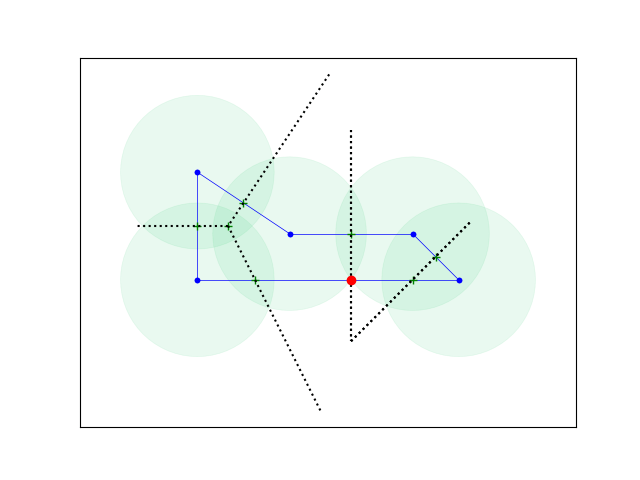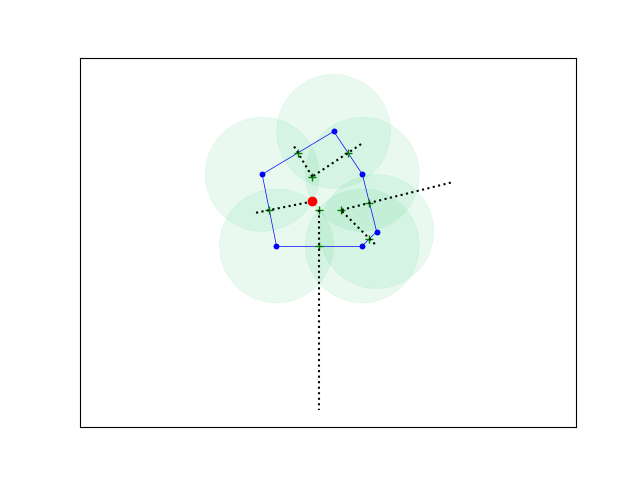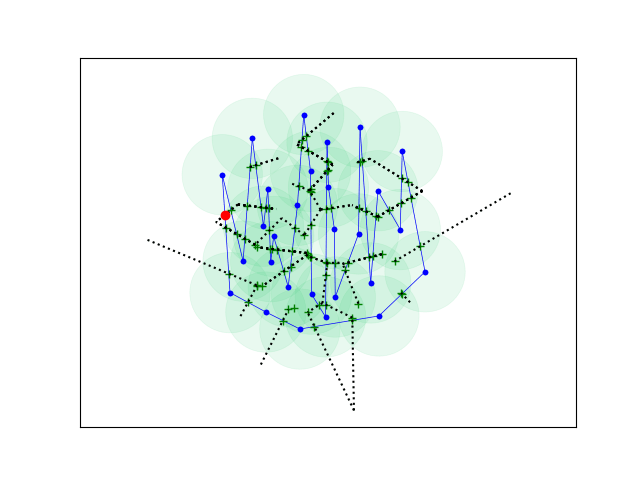I’m not sure if the solution point can be constructed directly any other way other than constructing the candidates efficiently and testing each one in turn. I’d be really interested to see if this problem can be solved any other way. This blog post was inspired by ACM ICPC World Finals 2018 Question G.